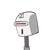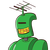# number of zeros of a polynomial of degree n is: equal to n, less than n, less than or equal to n, greater than n.

number of zeros of a polynomial of degree n is: equal to n, less than n, less than or equal to n, greater than n.

### 2 thoughts on “number of zeros of a polynomial of degree n is: equal to n, less than n, less than or equal to n, greater than n.”

1.less that or equal to n

Step-by-step explanation:

Example linear polynomial – degree 1 – roots 1

quadratic – degree 2 – roots 2 maximum

2.